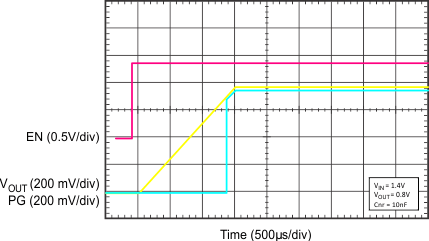SBVS197F May   2013  – October 2015

PRODUCTION DATA.

1. Features
2. Applications
3. Description
4. Revision History
5. Pin Configurations and Functions
6. Specifications
7. Detailed Description
1. 7.1 Overview
2. 7.2 Functional Block Diagram
3. 7.3 Feature Description
4. 7.4 Device Functional Modes
8. Application and Implementation
1. 8.1 Application Information
2. 8.2 Typical Application
3. 8.3 Do's and Don'ts
9. Power-Supply Recommendations
10. 10Layout
1. 10.1 Layout Guidelines
2. 10.2 Layout Example
11. 11Device and Documentation Support
1. 11.1 Device Support
1. 11.1.1 Development Support
2. 11.1.2 Device Nomenclature
2. 11.2 Documentation Support
3. 11.3 Community Resources
5. 11.5 Electrostatic Discharge Caution
6. 11.6 Glossary
12. 12Mechanical, Packaging, and Orderable Information

• RGR|20
• RGW|20
• RGR|20
• RGW|20

## 6 Specifications

### 6.1 Absolute Maximum Ratings

over junction temperature range (unless otherwise noted) (1)
MIN MAX UNIT
Voltage IN, BIAS, PG, EN –0.3 7.0 V
IN, BIAS, PG, EN (5% duty cycle) –0.3 7.5
SNS, OUT –0.3 VIN + 0.3 (2)
NR/SS, FB –0.3 3.6
50mV, 100mV, 200mV, 400mV, 800mV, 1.6V –0.3 VOUT + 0.3
Current OUT Internally limited A
PG (sink current into device) 5 mA
Operating junction temperature, TJ –55 150 °C
Storage temperature, Tstg –55 150 °C
(1) Stresses beyond those listed under Absolute Maximum Ratings may cause permanent damage to the device. These are stress ratings only, which do not imply functional operation of the device at these or any other conditions beyond those indicated under Recommended Operating Conditions. Exposure to absolute-maximum-rated conditions for extended periods may affect device reliability.
(2) The absolute maximum rating is VIN + 0.3 V or 7.0 V, whichever is smaller.

### 6.2 ESD Ratings

VALUE UNIT
V(ESD) Electrostatic discharge Human body model (HBM), per ANSI/ESDA/JEDEC JS-001(1) ±2000 V
Charged device model (CDM), per JEDEC specification JESD22-C101(2) ±500
(1) JEDEC document JEP155 states that 500-V HBM allows safe manufacturing with a standard ESD control process.
(2) JEDEC document JEP157 states that 250-V CDM allows safe manufacturing with a standard ESD control process.

### 6.3 Recommended Operating Conditions

over junction temperature range (unless otherwise noted)
MIN MAX UNIT
VIN Input supply voltage range 1.1 6.5 V
VBIAS Bias supply voltage range(1) 3.0 6.5 V
IOUT Output current 0 2 A
TJ Operating junction temperature –40 125 °C

### 6.4 Thermal Information

THERMAL METRIC(1) TPS7A8300 UNIT
RGW (QFN) RGR (QFN)
20 PINS 20 PINS
RθJA Junction-to-ambient thermal resistance 33.6 35.4 °C/W
RθJC(top) Junction-to-case (top) thermal resistance 30.0 47.6 °C/W
RθJB Junction-to-board thermal resistance 14.0 12.3 °C/W
ψJT Junction-to-top characterization parameter 0.2 0.5 °C/W
ψJB Junction-to-board characterization parameter 14.0 12.4 °C/W
RθJC(bot) Junction-to-case (bottom) thermal resistance 1.6 1.0 °C/W

### 6.5 Electrical Characteristics

Over operating temperature range (TJ = –40°C to 125°C), {1.1 V ≤ VIN < 1.4 V and 3.0 V ≤ VBIAS ≤ 6.5 V} or {VIN ≥ 1.4 V and VBIAS open}(1), VIN ≥ VOUT(TARGET) + 0.3 V(2), VOUT(TARGET) = 0.8 V, OUT connected to 50 Ω to GND(3), VEN = 1.1 V, COUT =
22 μF, CNR/SS = 0 nF, CFF = 0 nF, and PG pin pulled up to VIN with 100 kΩ, unless otherwise noted.
Typical values are at TJ = 25°C.
PARAMETER TEST CONDITIONS MIN TYP MAX UNIT
VIN Input supply voltage range 1.1 6.5 V
VBIAS Bias supply voltage range(1) 3.0 6.5 V
V(REF) Reference voltage V(REF) = V(FB) = V(NR/SS) 0.8 V
VUVLO1(IN) Input supply UVLO with BIAS VIN increasing 1.02 1.085 V
VHYS1(IN) VUVLO1(IN) hysteresis 320 mV
VUVLO2(IN) Input supply UVLO without BIAS VIN increasing 1.31 1.39 V
VHYS2(IN) VUVLO2(IN) hysteresis 253 mV
VUVLO(BIAS) Bias supply UVLO VBIAS increasing 2.83 2.9 V
VHYS(BIAS) VUVLO(BIAS) hysteresis 290 mV
VOUT Output voltage range Using voltage setting pins (50mV, 100mV, 200mV, 400mV, 800mV, and 1.6V) 0.8 – 1.0% 3.95 + 1.0% V
Using external resistors 0.8 – 1.0% 5.0 + 1.0%
Output voltage accuracy(4)(5) 0.8 V ≤ VOUT ≤ 5 V, 5 mA ≤ IOUT ≤ 2 A –1.0% 1.0%
VIN = 1.5 V, VOUT = 1.2 V, 5 mA ≤ IOUT ≤ 1.2 A –1.0% 1.0%
ΔVO(ΔVI) Line regulation IOUT = 5 mA, 1.4 V ≤ VIN ≤ 6.5 V 0.003 %/V
ΔVO(ΔIO) Load regulation 5 mA ≤ IOUT ≤ 2 A 0.0001 %/A
V(DO) Dropout voltage VIN ≥ 1.4 V and VBIAS open, 0.8 V ≤ VOUT ≤ 5.0 V,
IOUT = 2 A, VFB = 0.8 V – 3%
200 mV
VIN = 1.1 V, VBIAS = 5.0 V,
VOUT(TARGET) = 0.8 V, IOUT = 2 A, VFB = 0.8 V – 3%
125
I(LIM) Output current limit VOUT forced at 0.9 × VOUT(TARGET),
VIN = VOUT(TARGET) + 300 mV
2.1 3.4 4.2 A
I(GND) GND pin current Minimum load,
VIN = 6.5 V, no VBIAS supply, IOUT = 5 mA
2.8 4 mA
VIN = 1.4 V, no VBIAS supply, IOUT = 2 A
3.7 5
Shutdown, PG = (open),
VIN = 6.5 V, no VBIAS supply, V(EN) = 0.5 V
2.5 μA
I(EN) EN pin current VIN = 6.5 V, no VBIAS supply, V(EN) = 0 V and 6.5 V –0.1 0.1 μA
I(BIAS) BIAS pin current VIN = 1.1 V, VBIAS = 6.5 V,
VOUT(TARGET) = 0.8 V, IOUT = 2 A
2.3 3.5 mA
VIL(EN) EN pin low-level input voltage (disable device) 0 0.5 V
VIH(EN) EN pin high-level input voltage (enable device) 1.1 6.5 V
VIT(PG) PG pin threshold For the direction PG↓ with decreasing VOUT 0.82 VOUT 0.872 VOUT 0.93 VOUT V
Vhys(PG) PG pin hysteresis For PG↑ 0.02 VOUT V
VOL(PG) PG pin low-level output voltage VOUT < VIT(PG), IPG = –1 mA (current into device) 0.4 V
Ilkg(PG) PG pin leakage current VOUT > VIT(PG), V(PG) = 6.5 V 1 μA
I(NR/SS) NR/SS pin charging current VNR/SS = GND, VIN = 6.5 V 4.0 6.2 9.0 μA
IFB FB pin leakage current VIN = 6.5 V –100 100 nA
PSRR Power-supply ripple rejection f = 1 MHz, VIN = 3.8 V, VOUT = 3.3 V, IOUT = 2 A,
CNR/SS = 10 nF, CFF = 10 nF
40 dB
Vn Output noise voltage BW = 10 Hz to 100 kHz, VIN = 1.4 V, VOUT = 0.8 V,
IOUT = 1.5 A, CNR/SS = 10 nF, CFF = 10 nF
6 μVRMS
Tsd Thermal shutdown temperature Shutdown, temperature increasing 160 °C
Reset, temperature decreasing 140
TJ Operating junction temperature –40 125 °C
(1) BIAS supply is required when the VIN supply is below 1.4 V. Conversely, no BIAS supply is needed when the VIN supply is higher than or equal to 1.4 V.
(2) VOUT(TARGET) is the calculated VOUT target value from the output voltage setting pins: 50mV, 100mV, 200mV, 400mV, 800mV, and 1.6V in a fixed configuration. In an adjustable configuration, VOUT(TARGET) is the expected VOUT value set by the external feedback resistors.
(3) This 50-Ω load is disconnected when the test conditions specify an IOUT value.
(4) When the device is connected to external feedback resistors at the FB pin, external resistor tolerances are not included.
(5) The device is not tested under conditions where VIN > VOUT + 2.5 V and IOUT = 2 A, because the power dissipation is higher than the maximum rating of the package. Also, this accuracy specification does not apply on any application condition that exceeds the power dissipation limit of the package under test.

### 6.6 Typical Characteristics

At TJ = 25°C, {1.1 V ≤ VIN < 1.4 V and 3.0 V ≤ VBIAS ≤ 6.5 V} or {VIN ≥ 1.4 V and VBIAS open}
(1) BIAS supply is required when the VIN supply is below 1.4 V. Conversely, no BIAS supply is needed when the VIN supply is higher than or equal to 1.4 V.
, VIN ≥ VOUT(TARGET) + 0.3 V, VOUT(TARGET) = 0.8 V, OUT connected to 50 Ω to GND, VEN = 1.1 V, COUT = 22 μF, CNR/SS = 0 nF, CFF = 10 nF, and PG pin pulled up to VIN with 100 kΩ, unless otherwise noted.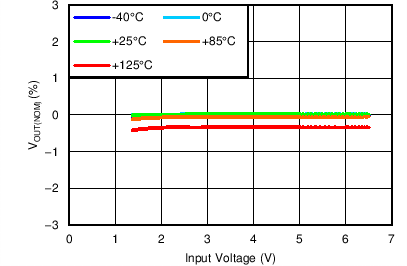VOUT(TARGET) = 0.8 V, IOUT = 5 mA, VBIAS = Open
Figure 1. Minimum ANY-OUT VOUT Line Regulation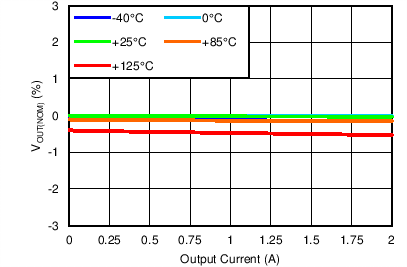VOUT(TARGET) = 0.8 V, VIN = 1.4 V, VBIAS = Open
Figure 3. Minimum ANY-OUT VOUT, Minimum VIN,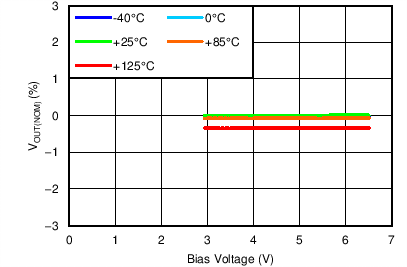VOUT(TARGET) = 0.8 V, VIN = 1.1 V, IOUT = 5 mA
Figure 5. Minimum ANY-OUT VOUT, Minimum VIN BIAS
Line Regulation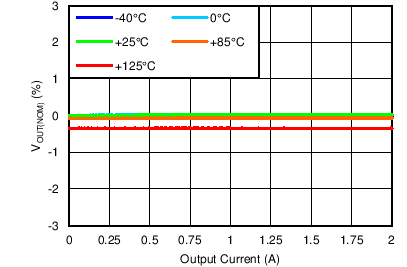VOUT(TARGET) = 0.8 V, VIN = 1.1 V, VBIAS = 6.5 V
Figure 7. Minimum Adjustable VOUT, Minimum VIN,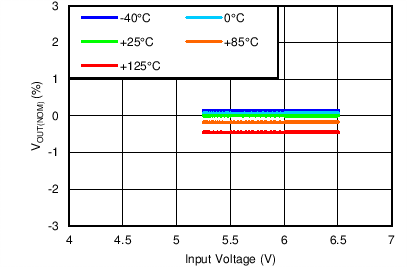VOUT(TARGET) = 5 V, IOUT = 5 mA, VBIAS = Open
Figure 9. Maximum Adjustable VOUT, No BIAS
Line Regulation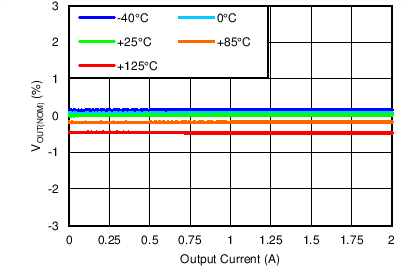VOUT(TARGET) = 5 V, VIN = 5.3 V, VBIAS = OpenVIN = 5.5 V, ANY-OUT, VBIAS = Open,
Figure 13. Dropout Voltage vs Output Current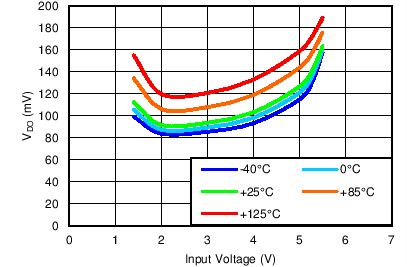VBIAS = Open, IOUT = 2 A, ANY-OUT
Figure 15. Dropout Voltage vs Input VoltageVIN = 1.1 V, ANY-OUT, IOUT = 2 A
Figure 17. Minimum VIN Dropout Voltage vs Bias Voltage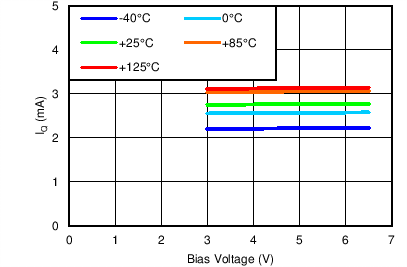VOUT(TARGET) = 0.8 V, IOUT = 5 mA, VIN = 1.1 V
Figure 19. Minimum ANY-OUT VOUT, Minimum VIN
Quiescent Current vs Bias VoltageVOUT(TARGET) = 0.8 V, VIN = 1.1 V, VBIAS = 3 V
Figure 21. Minimum ANY-OUT VOUT, VIN, and BIAS
Quiescent Current vs Output Current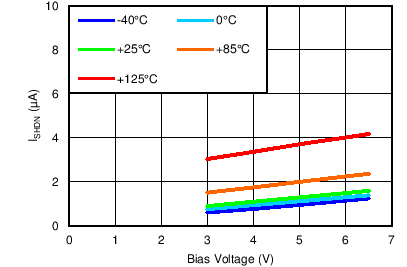VOUT(TARGET) = 0.8 V, VIN = 1.1 V
Figure 23. Minimum ANY-OUT VOUT, Minimum VIN
Shutdown Current vs Bias VoltageVIN = 1.8 V, ANY-OUT, VBIAS = Open, VOUT(TARGET) = 1.5 V
Figure 25. Current Limit vs Output Voltage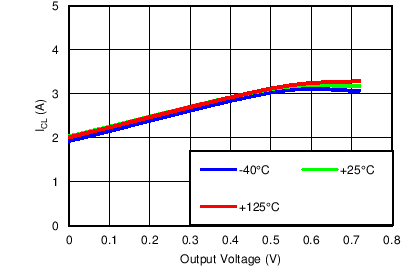VOUT(TARGET) = 0.8 V, VIN = 1.1 V, VBIAS = 3 V
Figure 27. Minimum ANY-OUT VOUT, VIN, and BIAS
Current Limit vs Output Voltage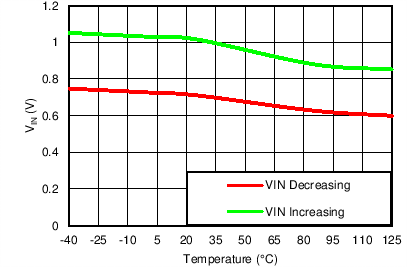VOUT(TARGET) = 0.8 V, VBIAS = 3.0 V
Figure 29. Minimum ANY-OUT VOUT, Minimum BIAS
Input UVLO Threshold vs Temperature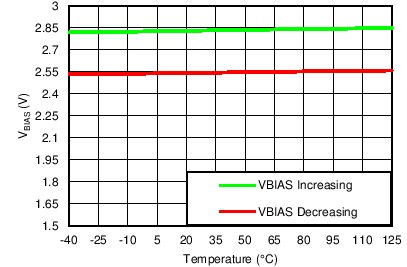VOUT(TARGET) = 0.8 V, VIN = 1.1 V
Figure 31. Minimum ANY-OUT VOUT, Minimum VIN BIAS
UVLO Threshold vs Temperature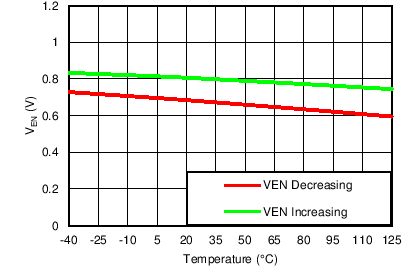VOUT(TARGET) = 0.8 V, VIN = 6.5 V, VBIAS = Open
Figure 33. Minimum ANY-OUT VOUT, Maximum VIN
Enable Threshold vs Temperature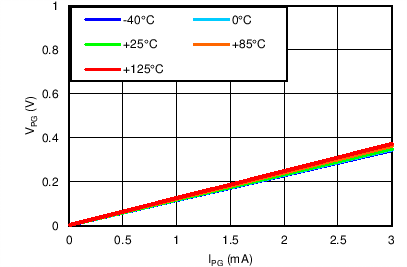VIN = 6.5 V, VOUT(TARGET) = 0.8 V, VBIAS = Open
Figure 35. Minimum ANY-OUT VOUT, Maximum VIN, No BIAS PG Low Voltage vs PG Current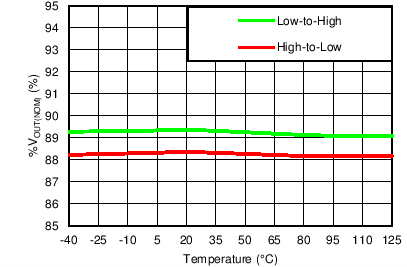VOUT(TARGET) = 0.8 V, VIN = 6.5 V, VBIAS = Open
Figure 37. Minimum ANY-OUT VOUT, Maximum VIN
PG Threshold vs Temperature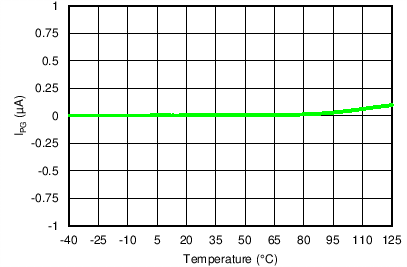VOUT(TARGET) = 0.8 V, VIN = VPG = 6.5 V, VBIAS = Open
Figure 39. Minimum ANY-OUT VOUT, Maximum VIN
PG Current vs Temperature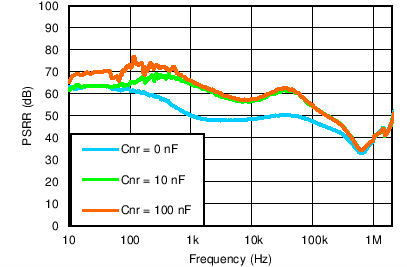VOUT(TARGET) = 3.3 V, ANY-OUT, VIN = VEN = 3.8 V, VBIAS = Open, IOUT = 1.5 A, COUT = 22 µF, CFF = 10 nF
Figure 41. Power-Supply Rejection vs CNR/SS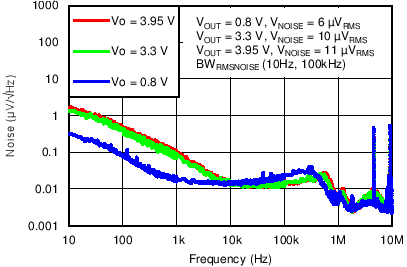VIN = VOUT(TARGET) + 0.5 V, ANY-OUT, VBIAS = Open, IOUT = 1.5 A, COUT = 22 µF, CNR/SS = CFF = 10 nF
Figure 43. Spectral Noise Density vs Output Voltage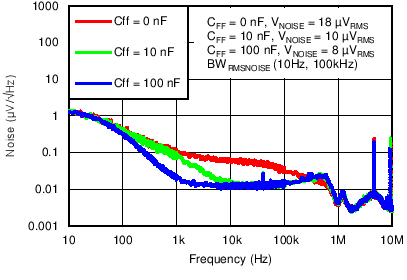VIN = 3.8 V, VOUT(TARGET) = 3.3 V, ANY-OUT, VBIAS = Open, IOUT = 1.5 A, COUT = 22 µF, CNR/SS = 10 nF
Figure 45. Spectral Noise Density vs CFF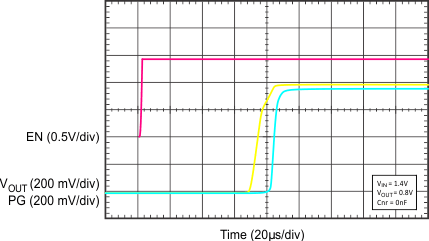VIN = 1.4 V, VOUT = 0.8 V, CNR/SS = 0 nF
Figure 47. Start-Up (CNR/SS = 0 nF)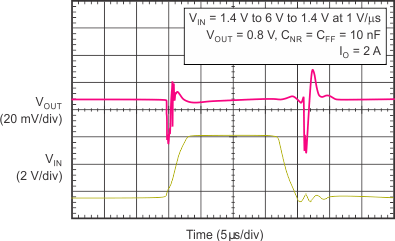VIN = 1.4 V to 6 V to 1.4 V at 1 V/µs, VOUT = 0.8 V, IOUT = 2 A, CNR/SS = CFF = 10 nF
Figure 49. Line Transient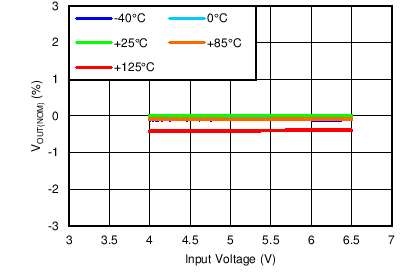VOUT(TARGET) = 3.95 V, IOUT = 5 mA, VBIAS = Open
Figure 2. Maximum ANY-OUT VOUT Line Regulation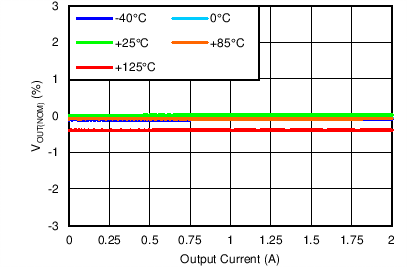VOUT(TARGET) = 3.95 V, VIN = 4.25 V, VBIAS = Open
Figure 4. Maximum ANY-OUT VOUT Load Regulation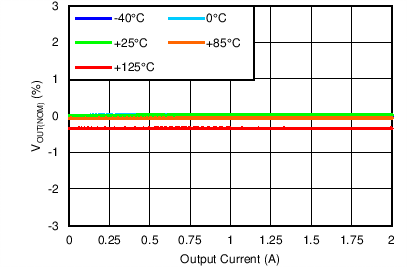VOUT(TARGET) = 0.8 V, VIN = 1.1 V, VBIAS = 3 V
Figure 6. Minimum ANY-OUT VOUT, VIN, and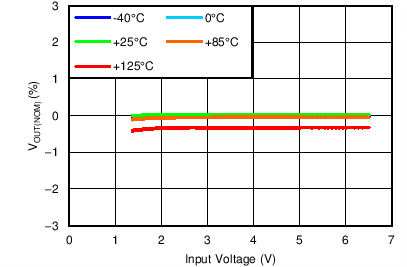VOUT(TARGET) = 0.8 V, IOUT = 5 mA, VBIAS = Open
Figure 8. Minimum Adjustable VOUT, No BIAS
Line Regulation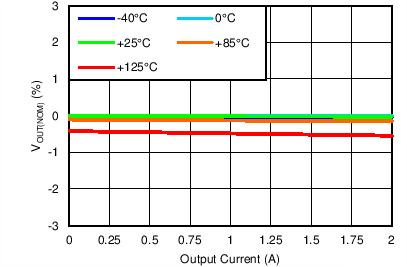VOUT(TARGET) = 0.8 V, VIN = 1.4 V, VBIAS = Open
Figure 10. Minimum Adjustable VOUT, Minimum VIN,VIN = 1.4 V, ANY-OUT, VBIAS = Open, No BIAS
Figure 12. Minimum VIN Dropout Voltage vs Output Current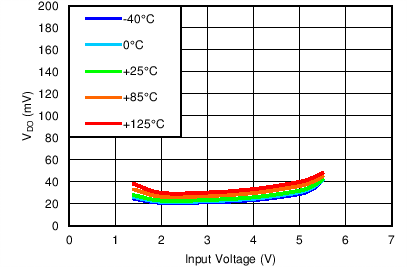VBIAS = Open, IOUT = 0.5 A, ANY-OUT
Figure 14. Dropout Voltage vs Input Voltage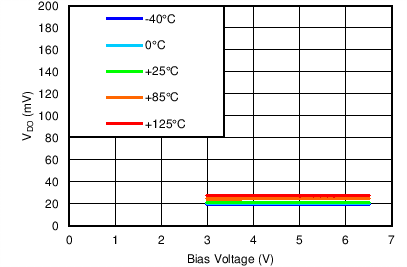VIN = 1.1 V, ANY-OUT, IOUT = 0.5 A
Figure 16. Dropout Voltage vs Bias Voltage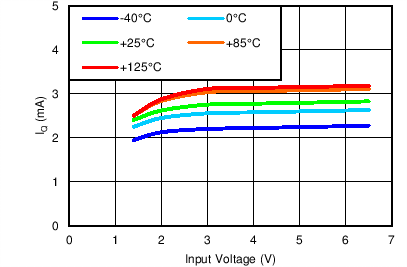VOUT(TARGET) = 0.8 V, IOUT = 5 mA, VBIAS = Open
Figure 18. Minimum ANY-OUT VOUT, No BIAS
Quiescent Current vs Input Voltage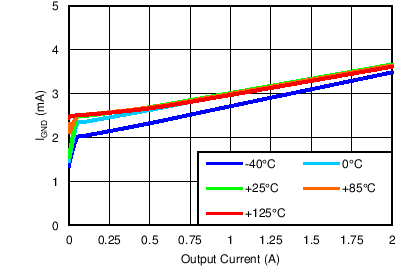VOUT(TARGET) = 0.8 V, VIN = 1.4 V, VBIAS = Open
Figure 20. Minimum ANY-OUT VOUT, Minimum VIN, No BIAS Quiescent Current vs Output Current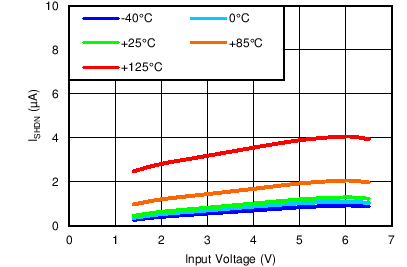VOUT(TARGET) = 0.8 V, VBIAS = Open
Figure 22. Minimum ANY-OUT VOUT, No BIAS
Shutdown Current vs Input Voltage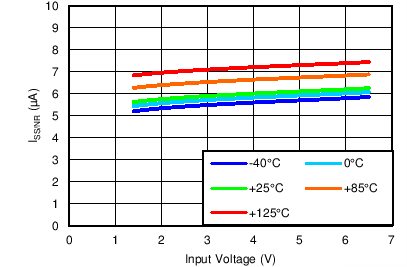VOUT(TARGET) = 0.8 V, VBIAS = Open
Figure 24. Minimum ANY-OUT VOUT, No BIAS
Soft-Start Current vs Input Voltage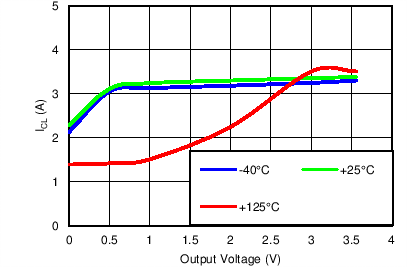VOUT(TARGET) = 3.95 V, VIN = 4.25 V, VBIAS = Open
Figure 26. Maximum ANY-OUT VOUT
Current Limit vs Output VoltageVOUT(TARGET) = 0.8 V, VIN = 1.1 V, VBIAS = 6.5 V
Figure 28. Minimum ANY-OUT VOUT, Minimum VIN,
Maximum BIAS Current Limit vs Output Voltage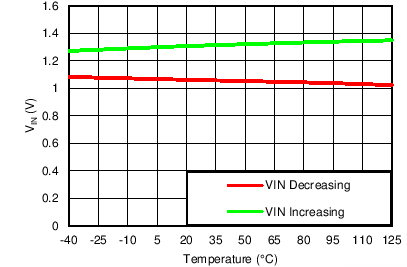VOUT(TARGET) = 0.8 V, VBIAS = Open
Figure 30. Minimum ANY-OUT VOUT, No BIAS
Input UVLO Threshold vs Temperature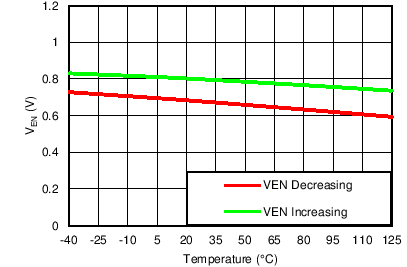VOUT(TARGET) = 0.8 V, VIN = 1.4 V, VBIAS = Open
Figure 32. Minimum ANY-OUT VOUT, Minimum VIN, No BIAS Enable Threshold vs Temperature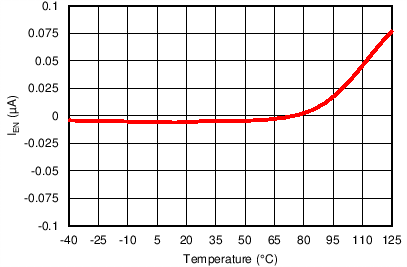VOUT(TARGET) = 0.8 V, VIN = VEN = 6.5 V, VBIAS = Open
Figure 34. Minimum ANY-OUT VOUT, Maximum VIN
Enable Current vs Temperature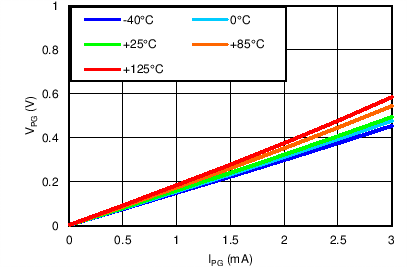VOUT(TARGET) = 0.8 V, VIN = 1.4 V, VBIAS = Open
Figure 36. Minimum ANY-OUT VOUT, Minimum VIN, No BIAS PG Low Voltage vs PG Current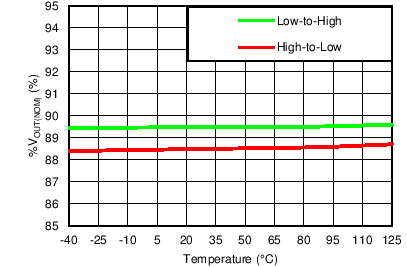VOUT(TARGET) = 0.8 V, VIN = 1.4 V, VBIAS = Open
Figure 38. Minimum ANY-OUT VOUT, Maximum VIN, No BIAS PG Threshold vs Temperature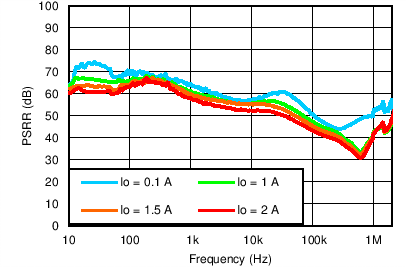VOUT(TARGET) = 3.3 V, ANY-OUT, VIN = VEN = 3.8 V, VBIAS = Open, COUT = 22 µF, CNR/SS = CFF = 10 nF
Figure 40. Power-Supply Rejection vs Output Current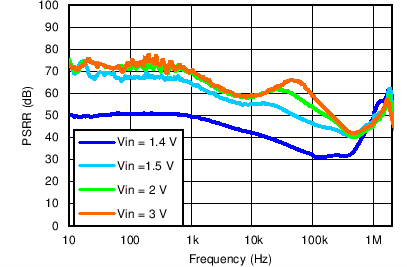VOUT(TARGET) = 1.2 V, ANY-OUT, VBIAS = Open, IOUT = 1.5 A, COUT = 22 µF, CNR/SS = CFF = 10 nF
Figure 42. Power-Supply Rejection vs Input Voltage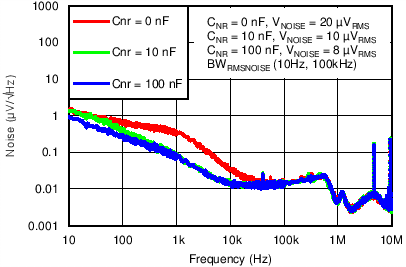VIN = 3.8 V, VOUT(TARGET) = 3.3 V, ANY-OUT, VBIAS = Open, IOUT = 1.5 A, COUT = 22 µF, CFF = 10 nF
Figure 44. Spectral Noise Density vs CNR/SSVIN = 3.85 V, VOUT = 3.3 V, IOUT = 100 mA to 1 A to 100 mA at 1 A/µs, CO = 22 µF